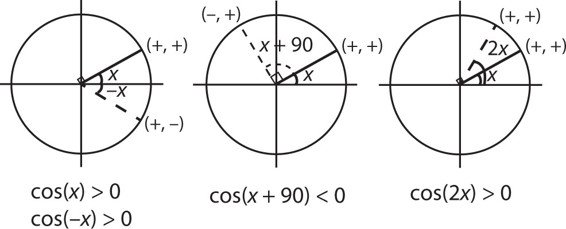# SAT Math Multiple Choice Question 281: Answer and Explanation

### Test Information

Question: 281

11. If x is an angle such that 0 < x < 90°, which of the following statements is not always true?

• A. cos(x) > 0
• B. cos(-x) > 0
• C. cos(x + 90°) < 0
• D. cos(2x) < 0

Explanation:

D

Difficulty: Hard

Category: Additional Topics in Math / Trigonometry

Strategic Advice: When a trig question involves signs (+ and -) rather than numbers, using your knowledge of how the unit circle works will get you to the answer. Before you begin analyzing the answer choices, translate the inequality symbols to signs (> 0 means +, and < 0 means -).

Getting to the Answer: Draw a quick sketch of a unit circle and add the angle x. Because 0 < x < 90°, you can draw the angle anywhere in the first quadrant. Drawing a small angle is usually the safest way to go.

Then, sketch in each of the angles described in the answers. Keep in mind that the cosine of the angle is equal to the x-coordinate of the point where the angle hits the unit circle, so it's positive in Quadrants I and IV and negative in Quadrants II and III.After sketching in each of the angles, compare them with the answer choices. The only one that is not always true is (D). When the angle is small, twice the angle still lands in Quadrant I, so the cosine is still positive (and therefore > 0, not < 0). Note that had you drawn a larger angle in Quadrant I, such as a 60° angle, 2x would have landed in Quadrant II, in which case the cosine would have been negative. However, the question asks for the statement that is not always true, so you must consider both large and small angles in Quadrant I.